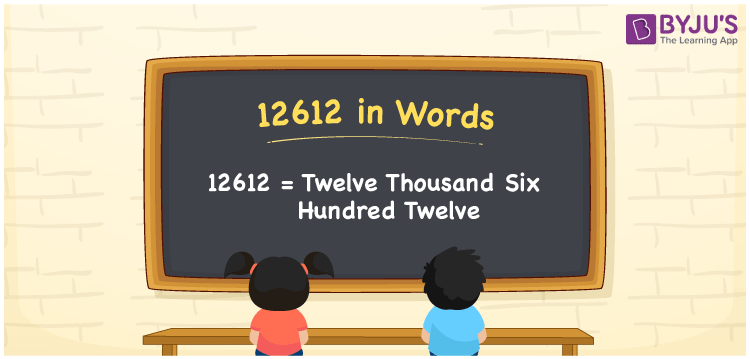# 12612 in Words

We can write 12612 in words as Twelve thousand six hundred twelve. As we know, 12612 is a cardinal number since it describes a specific quantity or amount. If you paid Rs. 12612 to buy a new chimney, you could say, “I paid Twelve thousand six hundred twelve rupees to purchase a new chimney”. Here, Twelve thousand six hundred twelve is the number name for 12612.

 12612 in words Twelve thousand six hundred twelve Twelve thousand six hundred twelve in Numbers 12612

## 12612 in English Words

Generally, we use the English alphabet to write numbers in words, Thus, we spell 12612 in English words as “Twelve thousand six hundred twelve”.## How to Write 12612 in Words?

Let’s learn how to write the number 12612 in words with the help of a five-column place value chart given below.

 Ten thousands Thousands Hundreds Tens Ones 1 2 6 1 2

Here, ones = 2, tens = 1, hundreds = 6, thousands = 2, ten thousands = 1

So, 1 × Ten thousand + 2 × Thousand + 6 × Hundred + 1 × Ten + 2 × One

= 1 × 10000 + 2 × 1000 + 6 × 100 + 1 × 10 + 2 × 1

= 10000 + 2000 + 600 + 10 + 2

= Ten Thousand + Two thousand + Six hundred + Ten + Two

= Twelve thousand + Six hundred + Twelve

= Twelve thousand six hundred twelve

Therefore, 12612 in words = Twelve thousand six hundred twelve.

12612 is a natural number that is the successor of 12611 and predecessor of 12613.

12612 in words – Twelve thousand six hundred twelve

Is 12612 an odd number? – No

Is 12612 an even number? – Yes

Is 12612 a prime number? – No

Is 12612 a composite number? – Yes

Is 12612 a perfect square number? – No

Is 12612 a perfect cube number? – No

## Frequently Asked Questions on 12612 in Words

### How do you write 12612 in English words?

We can write the number 12612 in words as Twelve thousand six hundred twelve.

### How do you write Rs. 12612 in words on a cheque?

On a cheque, we generally write Rs. 12612 in words as “Twelve thousand six hundred twelve rupees only”.

### Write the value of 12000 + 612 in words.

12000 + 612 = 12612 Thus, the value of 12000 + 612, i.e., 12612 in words is Twelve thousand six hundred twelve.